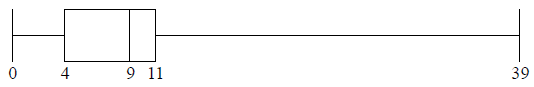# IB Math Analysis & Approaches Question bank-Topic: SL 4.3-Quartiles of discrete data SL Paper 1

## Question

A city hired 160 employees to work at a festival. The following cumulative frequency curve shows the number of hours employees worked during the festival.The city paid each of the employees £8 per hour for the first 40 hours worked, and £10 per hour for each hour they worked after the first 40 hours.

Find the median number of hours worked by the employees.


a.i.

Write down the number of employees who worked 50 hours or less.


a.ii.

Find the amount of money an employee earned for working 40 hours;


b.i.

Find the amount of money an employee earned for working 43 hours.


b.ii.

Find the number of employees who earned £200 or less.


c.

Only 10 employees earned more than £$$k$$. Find the value of $$k$$.


d.

## Markscheme

evidence of median position     (M1)

eg$$\,\,\,\,\,$$80th employee

40 hours     A1     N2

[2 marks]

a.i.

130 employees     A1     N1

[1 mark]

a.ii.

£320     A1     N1

[1 mark]

b.i.

splitting into 40 and 3     (M1)

eg$$\,\,\,\,\,$$3 hours more, $$3 \times 10$$

correct working     (A1)

eg$$\,\,\,\,\,$$$$320 + 3 \times 10$$

£350     A1     N3

[3 marks]

b.ii.

valid approach     (M1)

eg$$\,\,\,\,\,$$200 is less than 320 so 8 pounds/hour, $$200 \div 8,{\text{ }}25,{\text{ }}\frac{{200}}{{320}} = \frac{x}{{40}}$$,

18 employees     A2     N3

[3 marks]

c.

valid approach     (M1)

eg$$\,\,\,\,\,$$$$160 – 10$$

60 hours worked     (A1)

correct working     (A1)

eg$$\,\,\,\,\,$$$$40(8) + 20(10),{\text{ }}320 + 200$$

$$k = 520$$     A1     N3

[4 marks]

d.

## Question

The following box-and-whisker plot shows the number of text messages sent by students in a school on a particular day.Find the value of the interquartile range.


a.

One student sent k text messages, where k > 11 . Given that k is an outlier, find the least value of k.


b.

## Markscheme

recognizing Q1 or Q3 (seen anywhere)     (M1)

eg    4,11 , indicated on diagram

IQR = 7     A1 N2

[2 marks]

a.

recognizing the need to find 1.5 IQR     (M1)

eg   1.5 × IQR, 1.5 × 7

valid approach to find    (M1)

eg   10.5 + 11, 1.5 × IQR + Q3

21.5     (A1)

k = 22     A1 N3

Note: If no working shown, award N2 for an answer of 21.5.

[4 marks]

b.

## Question

A data set has n items. The sum of the items is 800 and the mean is 20.

The standard deviation of this data set is 3. Each value in the set is multiplied by 10.

Find n.


a.

Write down the value of the new mean.


b.i.

Find the value of the new variance.


b.ii.

## Markscheme

correct approach      (A1)

eg  $$\frac{{800}}{n} = 20$$

40      A1 N2

[2 marks]

a.

200   A1 N1

[1 mark]

b.i.

METHOD 1

recognizing variance = σ 2      (M1)

eg  32 = 9

correct working to find new variance      (A1)

eg  σ × 102, 9 × 100

900      A1 N3

METHOD 2

new standard deviation is 30      (A1)

recognizing variance = σ 2      (M1)

eg 32 = 9, 302

900      A1 N3

[3 marks]

b.ii.